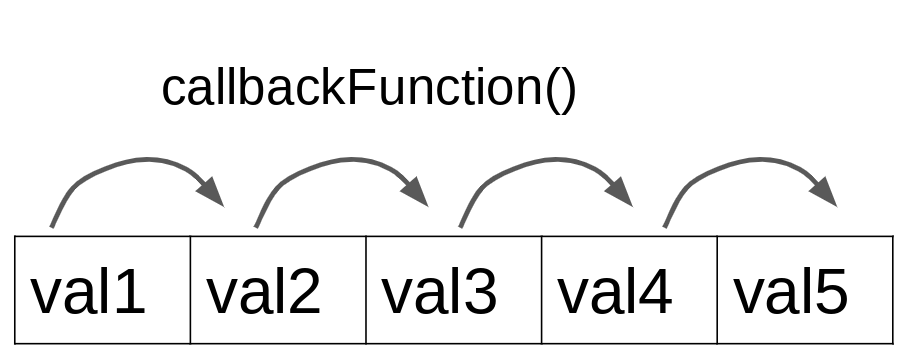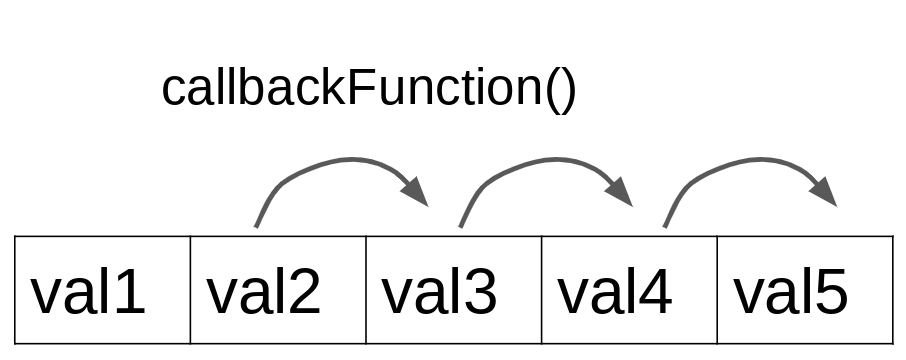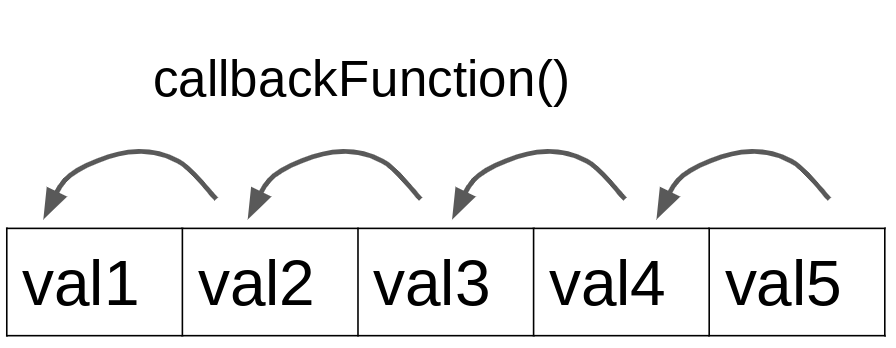# The Array methods reduce() and reduceRight() in Apps Script

The Array method `reduce()` reduces values in an array to a single value. The method reduceRight() does the same thing but it traverses the array from right to left. Aside from the order of traversal, both of these functions work in the same way.

Let us say we want to compute the sum of all numbers in the array `[1,2,3]`. You can use the `reduce()` method to do this since we're reducing the values in this array to a single value, their sum.

## Syntax

`array.reduce(callbackFunction, initialValue)`

## Parameters

callbackFunction (required):

• This function will be executed once for every element in the array. The output returned by each execution is passed as input to the next execution.

• The `callbackFunction` can accept four arguments:

• `previousOutput` (required): The output value returned by the previous execution.

• `value` (required): The current value being processed.

• `index` (optional): The index of the value in the array.

• `array` (optional): The original array that the `reduce()` method was called on.

initialValue (optional):

• If supplied, this initial value will be made available as the "previousOutput" when the callbackFunction is executed for the first element in the array. If it is not supplied, the first value in the array will be used as the "previousOutput" and the execution will begin

## Return value

The `reduce()` method "reduces'' an array to a single value. It executes the `callbackFunction` on every element of the original array. Each time, the output from the previous execution is made available to the current execution. The final output returned when the `callbackFunction` is executed for the last element in the array is returned by the `reduce()` method.

## How does the reduce() Array method work?

When the `reduce()` method is used on an array of values using a callback function, the callback function will be executed once for every value in the array. You can think of this as the callback function visiting each element of the array. In every visit, it remembers the output from its visit to the previous element in the array.

If you supply an initial value, the callback function will begin by visiting the first element in the array and the initial value will be treated as the previous output.If you do not supply an initial value, the callback function will begin by visiting the second element in the array and the first element will be treated as the previous output.In both of the above cases, the output from the first visit will be used as the previous output when the callback function visits the next element in the array. In this manner, the callback function is able to keep track of the updated output as it visits all the elements in the array. Finally, when it visits the last element in the array, its output from this final visit will be returned by the `reduce()` method.

### Illustrative example

Let us say we want to sum the values in the array `[1,2,3]` using the `reduce()` method. Consider the following function that we will use as the callback function. It accepts two parameters and returns their sum.

```function sum(previousOutput, currentValue) {
return previousOutput + currentValue;
}```

Suppose we do NOT provide an initial value while reducing the array, the callback function will begin by visiting the second element in the array and the first element will be used as the previous output.

```function sum(previousOutput, currentValue) {
return previousOutput + currentValue;
}

function reduceToSum() {
let values = [1,2,3];
return values.reduce(sum);
} ```

In this case, the execution will proceed as follows:

previousOutput

currentValue

What is executed?

output returned

Notes

1

2

sum(1,2)

3

Since no initial value was supplied, the callback function begins by visiting the second element in the array `[1,2,3]`, which is `2` and the first element (i.e. `1`) is treated as the previous output. The value `3` is returned.

3

3

sum(3,3)

6

The callback function moves on to the next element in the array, which is also the last element. The value `3` returned from the previous step is used as the previous output. The value `6` is returned. Since this is the last element, the `reduce()` method returns `6`.

Now, suppose we provide the initial value `0` while reducing the array. The callback function will begin by visiting the first element in the array and the initial value will be used as the previous output.

previousOutput

currentValue

What is executed?

output returned

Notes

0

1

sum(0,1)

1

Since `0` was supplied as the initial value, the callback function begins by visiting the first element in the array `[1,2,3]`, which is 1 and the initial value (i.e. `0`) is treated as the previous output. The `sum()` function is executed and the value `1` is returned.

1

2

sum(1,2)

3

The callback function moves on to the next element in the array, which is the second element. The value `1` returned from the previous step is used as the previous output. The `sum()` function is executed and the value `3` is returned.

3

3

sum(3,3)

6

The callback function moves on to the next element in the array, which is also the last element. The value `3` returned from the previous step is used as the previous output. The `sum()` function is executed and the value 6 is returned. Since this is the last element, the `reduce()` method returns 6.

In both cases, the array of values was reduced to the same output using the `sum()` callback function. However, the sequence of steps was different depending on whether an initial value was supplied or not.

### The reduceRight() method

The `reduceRight()` method works exactly like the `reduce()` method, except that it moves through an array from right to left versus left to right.## Examples

### Example 1: Using the reduce() Array method to sum values in an array.

#### Example 1.1: Sum values in an array without supplying an initial value

In the code below, the function `sum(previousValue, currentValue)` is the callback function that is supplied to the `reduce()` method. This function reduces the array of numbers to a single value, which is the sum of those values.

```function reduceEx1_1() {
var value = [1,2,3];
Logger.log(value.reduce(sum));
}

function sum(previousValue, currentValue) {
return previousValue + currentValue;
}```

Output

6.0

#### Example 1.2: Sum values in an array with supplying an initial value

Now, in addition to supplying a callback function, we will also supply an initial starting value. Since our goal is to sum the values in the array, we will supply an initial value of `0`: `value.reduce(sum, 0)`. Here, `sum` is the callbackFunction and `0` is the initial value. The output returned will be the same as in example 1.1 because adding the value `0` to the sum will not change the sum.

```function reduceEx1_2() {
var value = [1,2,3];
Logger.log(value.reduce(sum, 0));
}

function sum(previousValue, currentValue) {
return previousValue + currentValue;
}```

Output

6.0

### Example 2: Using the reduce() and reduceRight() Array methods to concatenate values in an array.

#### Example 2.1: Using the reduce() array method to concatenate values in an array

Consider the array of characters `["h", "e", "l", "l", "o"]`. The code below reduces these values to a single value by concatenating them together.

```function reduceEx2_1() {
var value = ["h", "e", "l", "l", "o"];
Logger.log(value.reduce(concat));
}

function concat(previousValue, currentValue) {
return previousValue + currentValue;
}```

Output

hello

#### Example 2.2: Using the reduceRight() array method to concatenate values in an array

Now, we'll do the same thing as example 2.1 except we will use the `reduceRight()` method instead of the `reduce()` method. Since the `reduceRight()` method moves from right to left, the order of characters in the concatenated string will be reversed.

```function reduceEx2_2() {
var value = ["h", "e", "l", "l", "o"];
Logger.log(value.reduceRight(concat));
}

function concat(previousValue, currentValue) {
return previousValue + currentValue;
}```

Output

olleh

## Conclusion

In this tutorial, you learned how to use the `reduce()` and `reduceRight()` Array methods. These methods reduce values in an array to a single value. The `reduce()` method moves through the array from left to right and the `reduceRight()` method moves from right to left.

## Stay up to date

Follow me via email to receive actionable tips and other exclusive content. I'll also send you notifications when I publish new content.